# 关于网络流f+f’也是原网络的一个可行流。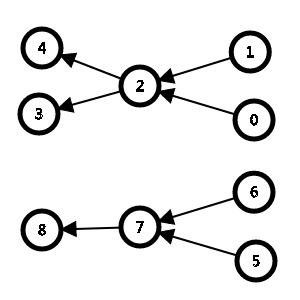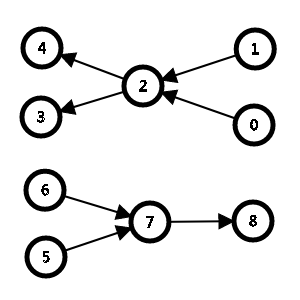| f + f’ | = | f | + | f’ |

1、割的容量：所有从S集合指向T集合的边的容量值和(顺序不能换），c(S,T)=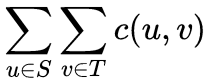最小割：割的最小容量

2.割的流量：从S指向T的流量-从T指向S的流量,即：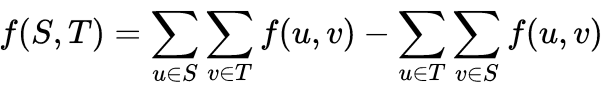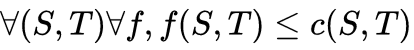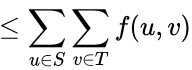$\because S\cup T = V,S\cap T = \phi$

$\therefore f(S,V)=f(S,S)+f(S,T)=0+f(S,T)$

$\therefore f(S,T)=f(S,V)=f(s,V)+f(S-s,V)$

$\because t\notin S,s\notin S-s$
$\therefore t,s\notin S-s$
$设S’=S-s$
$\therefore S’是一个不包括汇点源点的集合，他一定满足流量守恒$
$\therefore f(S’,V)=\sum_{u\in S’}^{}\sum_{v\in V}^{}f(u,v)- \sum_{u\in S’}^{}\sum_{v\in V}^{}f(v,u)$
$=\sum_{u\in S’}^{}(\sum_{v\in V}^{}f(u,v)-\sum_{v\in V}^{}f(v,u)$
$=\sum_{u\in S’}^{}(0)$
$=0$
$\therefore f(S,T)=f(s,T)$
$由定义得：f(s,V)=|f|$
$\therefore f(S,T)=|f|$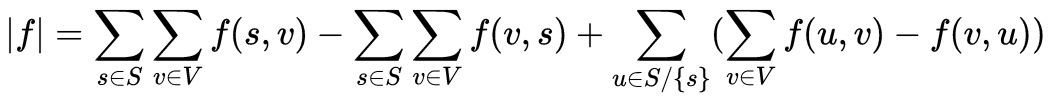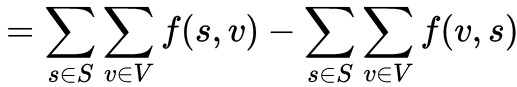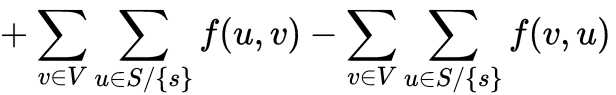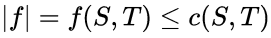即：最大流<=最小割